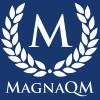# EV QuestionYour project team has completed 50% of works for a construction project with a total budget of \$ 1,250,000. So far the project has already spent \$ 400,000 and is expected to perform with the current rate until the end of the project. What is the correct statement about this project?

a. The project will finish within the schedule
b. The total cost of the project will be \$ 1,250,000
c. The project estimated requires \$ 400,000 to complete
d. The project sponsor concerns that the project might require to use the management reserves

### EV Question

Ans: B(it's not matching any of above options)

Explanation:

BAC: 1,250,000

EV: 50% of work=625000

AC=\$400,000.

EAC=BAC/CPI(Formila applied due to no variance)

=1,250,000/1.56

=801282

Already 400,000 spent, So  Total cost= 801282+400000=1201282### Use the full CPI results

Use the full CPI results

CPI = 625000 / 400000 = 1.5625

EAC = 1250000 / 1.5625 = 800000

ETC = 800000 - 400000 = 400000 (Answer C is correct)

### I believe the answer is A) Project will finish within schedule

First note - EV on this project is given as \$1,250,000/.50 =\$612,500

Note also: PV at the 50% stage is also \$612,500 i.e. 50% of BAC

Note also - SPI is Given by EV/PV

So SPI is exactly 1.0 - The project will finish exactly on schedule

B is wrong because our CPI = EV/AC = 612,500/400,000 = 1.5ish way ahead of cost which means we are on track to be way ahead of BAC of 1.25M.

C. Is wrong cos it just garbage.

My thought anyway.### Dear Brian, thanks for your

Dear Brian, thanks for your participation.

I'll try to discuss each answer.

Answer A : There's not enough information to state that this project will finish within the schedule. Even if there's a PV data (which is not available on the questions), it will not enough to reach that conclusion.

Answer B : The project is not finish yet, so we don't know the total project cost. At this stage, what we know is that \$1,250,000 is the project budget.

Aswer C :

EV = 625,000; AC = 400,000; BAC = 1,250,000

CPI = 625,000 / 400,000 = 1.5625

EAC = 1,250,000 / 1.5625 = 800,000

ETC = EAC - AC = 800,000 - 400,000 = 400,000

Answer D : As of now, the project performs well under budget, there's not enough information nor indication that suggest this project will went over budget and will need to use the management reserve.